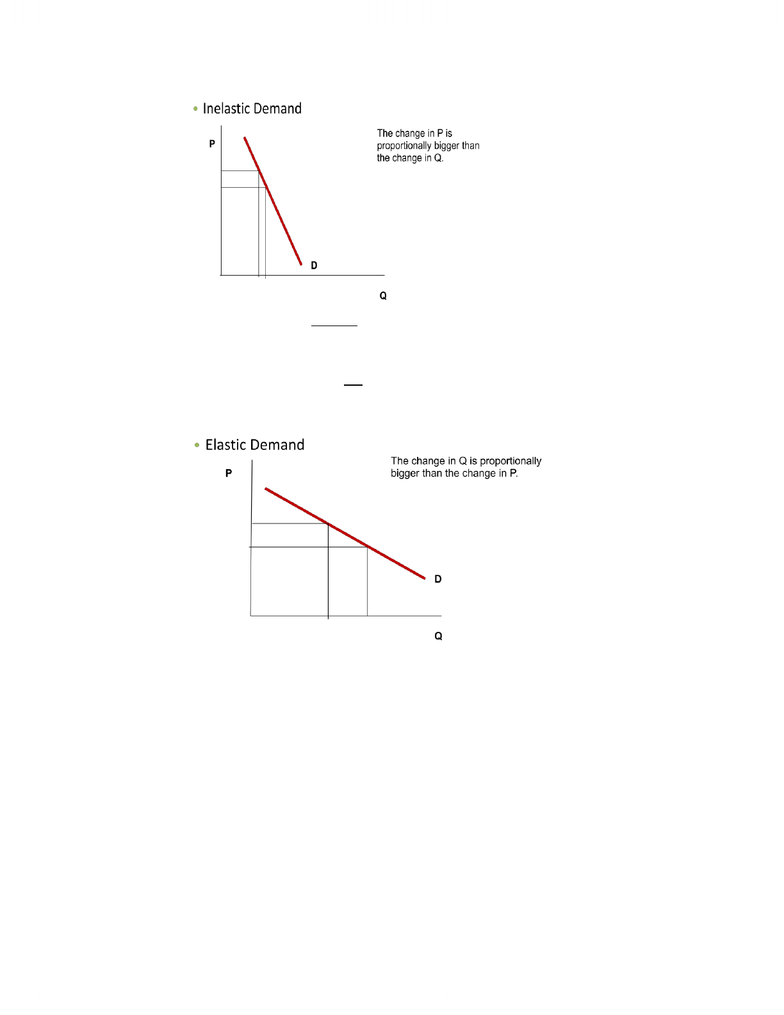Textbook Notes (280,000)
CA (160,000)
McMaster (10,000)
ECON (800)
ECON 1B03 (300)
Chapter 5

ECON 1B03 Chapter Notes - Chapter 5: Arc Elasticity, Demand Curve, List Of Bus Routes In Queens

Department
Economics
Course Code
ECON 1B03
Professor
Hannah Holmes
Chapter
5

This preview shows pages 1-2. to view the full 6 pages of the document.Thursday, January 24, 2013
Econ 1B03 Elasticity
Elasticity
Measures how responsive Qd or Qs is to change in price, income or prices of related goods.
o How much buyers and sellers respond to changes in market conditions?
Price elasticity of demand is a measure of how much the quantity demanded of a good responds
to a change in the price of that good
Elasticity of Demand
Price elasticity of demand is a measure of how much the quantity demanded of a good
responds to a change in the price of that good.
Price elasticity of demand is the percentage change in quantity demanded given a percent
change in the price.
The price elasticity of demand is computed as percentage change in quantity demanded divided
by percentage change in price
We’ll denote price elasticity by Ep.
Ep = percentage change in Qd / Percentage change in Price
Number we get from our calculations is called the coefficient of elasticity
The size of the coefficient, Ep, will tell us how elastic the good is how responsive demand is to
a change in price.
Since elasticity will vary, we can define different types of elasticity.
Types of Price Elasticity
People respond to changes in price differently depending on various factors.
Are there a large number of substitutes?
Is the good a luxury or necessity?
How narrowly defined is the market?
What about the time period?
Inelastic Demand: Quantity demanded does not respond strongly to price changes
o The % change in Qd < % change in P (The % change in Qd is not that big)
o If numerator is smaller than denominator our coefficient will be less than 1 (Ep < 1)
The demand curve would be fairly steep.
E.g required textbooks
o Change in P is proportionally bigger than change in Q, fairly steep

Only pages 1-2 are available for preview. Some parts have been intentionally blurred.Thursday, January 24, 2013
o
Elastic Demand: Qd responds strongly to changes in prices.
o % change in quantity demanded is proportionally greater than % change in Price
o Ep > 1
o Demand curve will be fairly flat
E.g most manufactures
o The change in Q is proportionally bigger than the change in P
o
Perfectly Inelastic Demand:
o Qd does not respond at all to a change in Price.
o Ep = 0
o The demand curve is completely vertical
E.g prescription heart medication
o Q doesn’t change for any size change in P.
You're Reading a Preview

Unlock to view full version